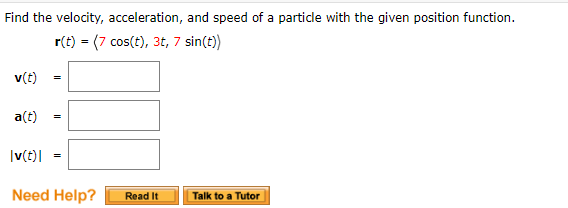Home / Answered Questions / Other / find-the-velocity-acceleration-and-speed-of-a-particle-with-the-given-position-function-r-t-7-cos-t--aw895

# (Solved): Find The Velocity, Acceleration, And Speed Of A Particle With The Given Position Function. R(t) = (7...Find the velocity, acceleration, and speed of a particle with the given position function. r(t) = (7 cos(t), 3t, 7 sin(t)) v(t) = a(t) = Iv(t) = Need Help? Read It Talk to a Tutor

We have an Answer from Expert